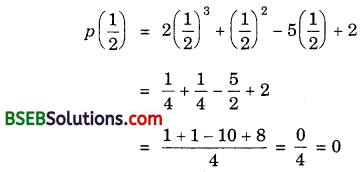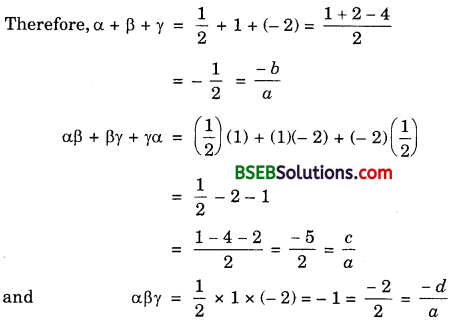# HSSlive: Plus One & Plus Two Notes & Solutions for Kerala State Board

## BSEB Class 10 Maths Chapter 2 Polynomials Ex 2.4 Textbook Solutions PDF: Download Bihar Board STD 10th Maths Chapter 2 Polynomials Ex 2.4 Book AnswersBSEB Class 10 Maths Chapter 2 Polynomials Ex 2.4 Textbook Solutions PDF: Download Bihar Board STD 10th Maths Chapter 2 Polynomials Ex 2.4 Book Answers

BSEB Class 10th Maths Chapter 2 Polynomials Ex 2.4 Textbooks Solutions and answers for students are now available in pdf format. Bihar Board Class 10th Maths Chapter 2 Polynomials Ex 2.4 Book answers and solutions are one of the most important study materials for any student. The Bihar Board Class 10th Maths Chapter 2 Polynomials Ex 2.4 books are published by the Bihar Board Publishers. These Bihar Board Class 10th Maths Chapter 2 Polynomials Ex 2.4 textbooks are prepared by a group of expert faculty members. Students can download these BSEB STD 10th Maths Chapter 2 Polynomials Ex 2.4 book solutions pdf online from this page.

## Bihar Board Class 10th Maths Chapter 2 Polynomials Ex 2.4 Books Solutions

 Board BSEB Materials Textbook Solutions/Guide Format DOC/PDF Class 10th Subject Maths Chapter 2 Polynomials Ex 2.4 Chapters All Provider Hsslive

## How to download Bihar Board Class 10th Maths Chapter 2 Polynomials Ex 2.4 Textbook Solutions Answers PDF Online?

2. Click on the Bihar Board Class 10th Maths Chapter 2 Polynomials Ex 2.4 Answers.
3. Look for your Bihar Board STD 10th Maths Chapter 2 Polynomials Ex 2.4 Textbooks PDF.
4. Now download or read the Bihar Board Class 10th Maths Chapter 2 Polynomials Ex 2.4 Textbook Solutions for PDF Free.

Find below the list of all BSEB Class 10th Maths Chapter 2 Polynomials Ex 2.4 Textbook Solutions for PDF’s for you to download and prepare for the upcoming exams:

## BSEB Bihar Board Class 10th Maths Solutions Chapter 2 Polynomials Ex 2.4

Question 1.
Verify that the numbers given alongside of the cubic polynomials below are their zeros.
Also verify the relationship between the zeroes and the coefficients in each case:

1. 2x3 + x2 – 5x + 2; 12, 1, – 2
2. x3 – 4x2 + 5x – 2; 2, 1, 1

Solution:
1. Comparing the given polynomial with ax3 + bx2 + cx + d, we get
a = 2, b = 1, c = – 5 and d = 2.p(1) = 2(1)3 + (1)2 – 5(1) + 2
= 2 + 1 – 5 + 2 = 0
p(- 2) = 2(- 2)3 + (- 2)2 – 5(- 2) + 2
= 2(- 8) + 4 + 10 + 2
= – 16 + 16 = 0
∴ 12, 1 and – 2 are the zeroes of 2x3 + x2 – 5x + 2.
So, α = 12, β = 1 and γ = – 2.2. Comparing the given polynomial with ax3 + bx2 + cx + d, we get
a = 1, b = – 4, c = 5 and d = – 2.
p(2) = (2)3 – 4(2)2 + 5(2) – 2
= 8 – 16 + 10 – 2 = 0
p(1) = (1)3 – 4(1)2 + 5(1) – 2
= 1 – 4 + 5 – 2 = 0
∴ 2, 1 and 1 are the zeroes of x3 – 4x2 + 5x – 2.
So, α = 2, β = 1 and γ = 1.
Therefore, α + β + γ = 2 + 1 + 1 = 4 = −(−4)1 = −𝑏𝑎
αβ + βγ + γα = (2)(1) + (1)(1) + (1)(2)
= 2 + 1 + 2 + 5 = 51 = 𝑐𝑎
and αβγ = (2)(1)(1) = 2 = −(−2)1 = −𝑑𝑎.

Question 2.
Find a cubic polynomial with the sum, sum of the products of its zeroes taken two at a time, and the product of its zeroes as 2, – 7, – 14 respectively.
Solution:
Let the cubic polynomial be ax3 + bx2 + cx + d, and its zeroes be α, β and γ.
Then, α + β + γ = 2 = −(−2)1 = −𝑏𝑎
αβ + βγ + γα = – 7 = −71 = 𝑐𝑎
and αβγ = – 14 = −141 = −𝑑𝑎
If a = 1, then b = – 2, c = – 7 and d = 14.
So, one cubic polynomial which fits the given conditions x3 – 2x2 – 7x + 14.

Question 3.
If the zeroes of the polynomial x3 – 3x2 + x + 1 are a – b, a and a + b, find a and b.
Solution:
Since (a – b), a and (a + b) are the zeroes of the polynomial x3 – 3x2 + x + 1, therefore
So, (a – b) + a + (a + b) = −(−3)1 = 3
So, 3a = 3 or a = 1
(a – b)a + a(a + b) + (a + b)(a – b) = 11 = 1
or a2 – ab + a2 + ab + a2 – b2 = 1
or 3a2 – b2 = 1
So, 3(1)2 – b2 = 1 [∵ a = 1]
or 3 – b2 = 1
or b2 = 2 or b = ± 2‾√
Hence, a = 1 and b = ± 2‾√.

Question 4.
If two zeroes of the polynomial x4 – 6x3 – 26x2 + 138x – 35 are 2 ± 3‾√, find other zeroes.
Solution:
We have:
2 ± 3‾√ are two zeroes of the polynomial p(x) = x4 – 6x3 – 26x2 + 138x – 35
Let x = 2 ± 3‾√. So, x – 2 = ± 3‾√
Squaring, we get
x2 – 4x + 4 = 3, i.e; x2 – 4x + 1 = 0
Let us divide p(x) by x2 – 4x + 1 to obtain other zeroes.∴ p(x) = x4 – 6x3 – 26x2 + 138x – 35
= (x2 – 4x + 1)(x2 – 2x – 35)
= (x2 – 4x + 1)(x2 – 7x + 5x – 35)
= (x2 – 4x + 1)[x(x – 7) + 5(x – 7)]
= (x2 – 4x + 1)(x + 5)(x – 7)
So, (x + 5) and (x – 7) are other factors of p(x).
∴ – 5 and 7 are other zeroes of the given polynomial.

Question 5.
If the polynomial x4 – 6x3 + 16x2 – 25x + 10 is divided by another polynomial x2 – 2x + k, the remainder comes out to be x + a, find k and a.
Solution:
Let us divide x4 – 6x3 + 16x2 – 25x + 10 by x2 – 2x + k.∴ Remainder = (2k – 9)x – (8 – k)k + 10
But the remainder is given as x + a.
On comparing their coefficients, we have:
2k – 9 = 1 or 2k = 10 or k = 5
and – (8 – k)k + 10 = a
So, a = – (8 – 5)5 + 10
= – 3 × 5 + 10 = – 15 + 10 = – 5
Hence, k = 5 and a = – 5.

## Bihar Board Class 10th Maths Chapter 2 Polynomials Ex 2.4 Textbooks for Exam Preparations

Bihar Board Class 10th Maths Chapter 2 Polynomials Ex 2.4 Textbook Solutions can be of great help in your Bihar Board Class 10th Maths Chapter 2 Polynomials Ex 2.4 exam preparation. The BSEB STD 10th Maths Chapter 2 Polynomials Ex 2.4 Textbooks study material, used with the English medium textbooks, can help you complete the entire Class 10th Maths Chapter 2 Polynomials Ex 2.4 Books State Board syllabus with maximum efficiency.

## FAQs Regarding Bihar Board Class 10th Maths Chapter 2 Polynomials Ex 2.4 Textbook Solutions

#### Can we get a Bihar Board Book PDF for all Classes?

Yes you can get Bihar Board Text Book PDF for all classes using the links provided in the above article.

## Important Terms

Bihar Board Class 10th Maths Chapter 2 Polynomials Ex 2.4, BSEB Class 10th Maths Chapter 2 Polynomials Ex 2.4 Textbooks, Bihar Board Class 10th Maths Chapter 2 Polynomials Ex 2.4, Bihar Board Class 10th Maths Chapter 2 Polynomials Ex 2.4 Textbook solutions, BSEB Class 10th Maths Chapter 2 Polynomials Ex 2.4 Textbooks Solutions, Bihar Board STD 10th Maths Chapter 2 Polynomials Ex 2.4, BSEB STD 10th Maths Chapter 2 Polynomials Ex 2.4 Textbooks, Bihar Board STD 10th Maths Chapter 2 Polynomials Ex 2.4, Bihar Board STD 10th Maths Chapter 2 Polynomials Ex 2.4 Textbook solutions, BSEB STD 10th Maths Chapter 2 Polynomials Ex 2.4 Textbooks Solutions,
Share: